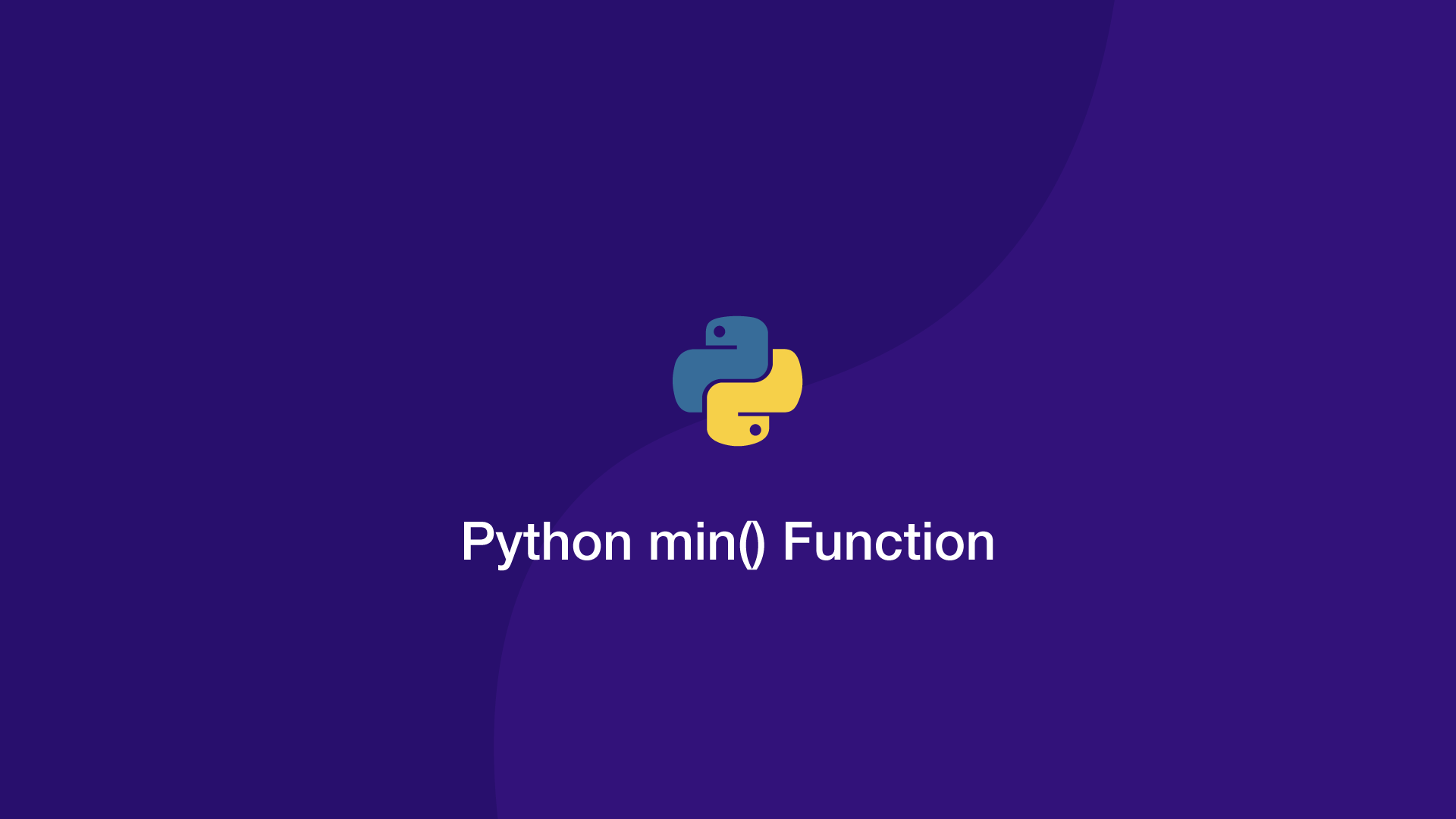# How to use the Python min() FunctionThe `min()` Function in Python returns the smallest value from an iterable.

Before we look at some examples to demonstrate how this function behaves, let's look at the syntax of `min()`.

## The min() Syntax

`min()` has one required argument, the iterable and two optional arguments, the default value and sort key.

``````min(iterable[, default=object, key=function])
``````

• `iterable` – the iterable to sort.
• `default` – a default value to return if the iterable is empty.
• `key` – a function to use for sorting the iterable in a different way.

## Get Smallest Value in a List in Python

To begin, let's look at how we would use the Python `min()` function to get the smallest value from a list of integers.

``````numbers = [2,3,1,6,9,8]

result = min(numbers)

print(result)
``````
``````1
``````

1 is the smallest in the above list and that is indeed what we got back.

Letters also have a hierarchy of value, with A being the lowest and z being the highest (all uppercase letters have a lower value than lowercase ones.)

``````string = 'Yaz'

result = min(string)

print(result)
``````
``````Y
``````

## Change min() Sort Order

Let's say we have a list of mixed case letters but we don't want to count the case as being a ranking factor. We can use a function inside the `key=` argument to turn each letter to lowercase before it is evaluated like this:

``````string = 'Yaz'

result = min(string, key=str.lower)

print(result)
``````
``````a
``````

## Get Shortest Element in Python

Another useful way to use the `key=` argument inside the `min()` argument is for getting the shortest element in a list by its length like this:

``````items = ['orange', 'apple', 'strawberry']

shortest = min(items, key=len)

print(shortest)
``````
``````apple
``````

#### Related Tutorials### How to Calculate Mean, Median, Mode and Range in Python

October 07, 2020### How to Generate Random Numbers in JavaScript

September 13, 2020### How to Get a Rolling Mean From a pandas DataFrame in Python

May 25, 2021### How to use Sets in Python

September 15, 2020### Convert Unix Epoch/Timestamp to Datetime in Python

July 09, 2021August 30, 2020